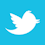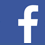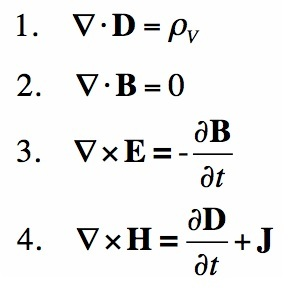The famous mathematician can guide our understanding of electrical signals.

Maxwell’s famous equations describing electricity were published in 1868. Many historians feel this is the greatest contribution ever made to science. Maxwell had none of the components or instruments we know today. The fact that these equations agree with relativity theory is even more amazing. These equations are field-based because that is the side of nature that could be observed. The genius was to express these field ideas as concise differential equations.

Many electronic engineers leave Maxwell to the textbooks and use circuit theory and lore to design circuits. In logic, the rise times for signals are often less than a ns. This represents bandwidth that invites unwanted radiation, interference and crosstalk. Maxwell’s equations provide explanations for all these mechanisms. I feel solving difficult mathematical equations is not usually the right way to make progress. As an example, it is far more important to know how delays occur than to calculate the exact delay. It is more important to know where energy is lost than to calculate the actual losses. There is a lot to be gained by looking at what Maxwell can tell us.

The movement of information on transmission lines is usually the movement of energy in the capacitance and inductance located between traces and ground planes. It is important to realize these spaces must be designed if problems are to be avoided. Letting signals share spaces invites problems. Wave action does not usually reflect energy as is commonly thought. I will comment on this fact later.FIGURE 1. Maxwell's equations of the behavior of electric and magnetic fields. (Source: maxwells-equations.com)

##### Fields

A parameter that has intensity and direction at all points in space is called a field. There are two electric and two magnetic field measures used in Maxwell’s equations. If you sense a voltage difference in a circuit, you are observing the presence of an E or electric field. If you sense current flow, you are observing the presence of an H or magnetic field. Depending on the materials involved, the field patterns show how much electromagnetic energy is stored or is being moved.1

If a switch makes a change to a circuit storing field energy, electric and magnetic fields acting together will allow energy to reconfigure in the spaces between conductors or perhaps radiate energy from an antenna. This is nature taking every opportunity to spread potential energy into available spaces. A simple example is a battery switched to a pair of conductors that we call a transmission line. This connection will allow nature to move electric and magnetic field energy in a new space. If the result is a new stable voltage at a logic gate, we have performed a logic function. To return the logic to its first state, any energy added to the system must be dissipated or radiated.

##### The First Wave on a Transmission Line

The transmission line we will examine first is a trace over a ground plane. Assume the voltage source is a ramp voltage with a rise time of 0.1ns. The rising voltage causes a current flow and an associated magnetic field along the line. The combination of electric and magnetic field carries energy forward at the speed of light. The charges associated with the E and H fields move in the conductors at centimeters per second. In a practical sense, the only thing moving is energy at the speed of light. We have no energy meters, so we must learn how to predict nature’s actions for useful engineering.

When the voltage wave starts forward in a transmission line, current starts to flow on the trace. Actually, the current flows in a loop that moves with the changing electric field. A changing E field (a rising voltage) is a static H field. This is one of Maxwell’s equations. A static H field is equal to a constant current. This is the loop current that returns to the voltage source via the ground plane and charges the transmission line capacitance to the peak of the wave’s leading-edge voltage. This leading-edge voltage travels at the speed of light.

The essence of this process is the electric and magnetic fields are static behind the wave front, and yet energy is moving forward at the speed of light. The idea that energy moves rapidly and the parameters of energy motion are static is not new. The driveshaft of an electric generator carries energy forward at a high velocity, but the parameters are rpm and stress that do not move forward. Notice that in a flashlight the electric and magnetic fields are constant, yet energy moves at the speed of light.

##### Reflections at an Open Circuit

The energy that moves in a transmission line moves in the space between conductors. On the leading edge, energy is in transition. In the first wave, energy is moved into the capacitance of the line. If the rise time is long enough or if the line is short enough there will be no reflection when the wave reaches an open circuit. I want to discuss the short rise time case where there is a reflection at an open circuit. When this first wave reaches the end of the line, energy continues to flow into the line capacitance. There is no mechanism that stops this forward energy flow. The result is a reflected wave that doubles the voltage along the line. Behind the first reflected wave there is no current flow. When the first reflected wave reaches the source, the line is charged to twice the voltage source and the current flow is zero along the entire line. The next reflection must return the line voltage to its initial value. The energy for this wave is half the stored electric field. The conversion of half the E field to H field is described by Maxwell’s equations. From this point on, wave action converts electric to magnetic field energy in an oscillating manner. This is similar to a swinging pendulum that is constantly converting energy. Half the time energy is moving to the E field and half the time it is moving to the H field. The only time energy is radiated is on the leading edge where field energy is in transition.

I have written a book titled Fast Circuit Boards – Energy Management published by John Wiley. It discusses transmission lines and moving energy in detail. Topics include gap and via placement, decoupling capacitors and power planes. In the long run, progress in electronics depends on compromises and an understanding between users and component designers. I know from experience that change across disciplines can be difficult and slow. It is important to accept field ideas because this is where the future lies. Progress and basic understanding go together.

Buildings have walls and halls

People walk in halls not walls

Circuits have traces and spaces

Energy uses spaces not traces

##### Notes

1. The second electric field mentioned in Maxwell’s equations is the D or displacement field. In statics the D field starts and ends on charges. The value of D = ε0εRE where E is the electric field, ε0 is the permittivity of free space and εis the relative dielectric constant. The second measure of the magnetic field mentioned in Maxwell’s equations is the B or induction field. The value of B = μR μ0 H where μis the relative permeability of the material and μ0 is the permeability of free space.

Ralph Morrison has 50 years of experience in electronics engineering and is author of eight books, including Solving Interference Problems in Electronics, Grounding and Shielding Techniques in Instrumentation, and The Fields of Electronics: Understanding Electronics Using Basic Physics; This email address is being protected from spambots. You need JavaScript enabled to view it..